# WebCam Motion Detector in Python

This python program will allow you to detect motion and also store the time interval of the motion.

Requirement:

1. Python3
2. OpenCV(libraries)
3. Pandas(libraries)

Install Requirments : Install Python3, install Pandas and OpenCV libraries.

Main Logic : Videos can be treated as stack of pictures called frames. Here i am comparing different frames(pictures) to the first frame which should be static(No movements initially). We compare two images by comparing the intensity value of each pixels. In python we can do it easily as you can see in following code:

 `# Python program to implement  ` `# WebCam Motion Detector ` ` `  `# importing OpenCV, time and Pandas library ` `import` `cv2, time, pandas ` `# importing datetime class from datetime library ` `from` `datetime ``import` `datetime ` ` `  `# Assigning our static_back to None ` `static_back ``=` `None` ` `  `# List when any moving object appear ` `motion_list ``=` `[ ``None``, ``None` `] ` ` `  `# Time of movement ` `time ``=` `[] ` ` `  `# Initializing DataFrame, one column is start  ` `# time and other column is end time ` `df ``=` `pandas.DataFrame(columns ``=` `[``"Start"``, ``"End"``]) ` ` `  `# Capturing video ` `video ``=` `cv2.VideoCapture(``0``) ` ` `  `# Infinite while loop to treat stack of image as video ` `while` `True``: ` `    ``# Reading frame(image) from video ` `    ``check, frame ``=` `video.read() ` ` `  `    ``# Initializing motion = 0(no motion) ` `    ``motion ``=` `0` ` `  `    ``# Converting color image to gray_scale image ` `    ``gray ``=` `cv2.cvtColor(frame, cv2.COLOR_BGR2GRAY) ` ` `  `    ``# Converting gray scale image to GaussianBlur  ` `    ``# so that change can be find easily ` `    ``gray ``=` `cv2.GaussianBlur(gray, (``21``, ``21``), ``0``) ` ` `  `    ``# In first iteration we assign the value  ` `    ``# of static_back to our first frame ` `    ``if` `static_back ``is` `None``: ` `        ``static_back ``=` `gray ` `        ``continue` ` `  `    ``# Difference between static background  ` `    ``# and current frame(which is GaussianBlur) ` `    ``diff_frame ``=` `cv2.absdiff(static_back, gray) ` ` `  `    ``# If change in between static background and ` `    ``# current frame is greater than 30 it will show white color(255) ` `    ``thresh_frame ``=` `cv2.threshold(diff_frame, ``30``, ``255``, cv2.THRESH_BINARY)[``1``] ` `    ``thresh_frame ``=` `cv2.dilate(thresh_frame, ``None``, iterations ``=` `2``) ` ` `  `    ``# Finding contour of moving object ` `    ``(_, cnts, _) ``=` `cv2.findContours(thresh_frame.copy(),  ` `                       ``cv2.RETR_EXTERNAL, cv2.CHAIN_APPROX_SIMPLE) ` ` `  `    ``for` `contour ``in` `cnts: ` `        ``if` `cv2.contourArea(contour) < ``10000``: ` `            ``continue` `        ``motion ``=` `1` ` `  `        ``(x, y, w, h) ``=` `cv2.boundingRect(contour) ` `        ``# making green rectangle arround the moving object ` `        ``cv2.rectangle(frame, (x, y), (x ``+` `w, y ``+` `h), (``0``, ``255``, ``0``), ``3``) ` ` `  `    ``# Appending status of motion ` `    ``motion_list.append(motion) ` ` `  `    ``motion_list ``=` `motion_list[``-``2``:] ` ` `  `    ``# Appending Start time of motion ` `    ``if` `motion_list[``-``1``] ``=``=` `1` `and` `motion_list[``-``2``] ``=``=` `0``: ` `        ``time.append(datetime.now()) ` ` `  `    ``# Appending End time of motion ` `    ``if` `motion_list[``-``1``] ``=``=` `0` `and` `motion_list[``-``2``] ``=``=` `1``: ` `        ``time.append(datetime.now()) ` ` `  `    ``# Displaying image in gray_scale ` `    ``cv2.imshow(``"Gray Frame"``, gray) ` ` `  `    ``# Displaying the difference in currentframe to ` `    ``# the staticframe(very first_frame) ` `    ``cv2.imshow(``"Difference Frame"``, diff_frame) ` ` `  `    ``# Displaying the black and white image in which if ` `    ``# intencity difference greater than 30 it will appear white ` `    ``cv2.imshow(``"Threshold Frame"``, thresh_frame) ` ` `  `    ``# Displaying color frame with contour of motion of object ` `    ``cv2.imshow(``"Color Frame"``, frame) ` ` `  `    ``key ``=` `cv2.waitKey(``1``) ` `    ``# if q entered whole process will stop ` `    ``if` `key ``=``=` `ord``(``'q'``): ` `        ``# if something is movingthen it append the end time of movement ` `        ``if` `motion ``=``=` `1``: ` `            ``time.append(datetime.now()) ` `        ``break` ` `  `# Appending time of motion in DataFrame ` `for` `i ``in` `range``(``0``, ``len``(time), ``2``): ` `    ``df ``=` `df.append({``"Start"``:time[i], ``"End"``:time[i ``+` `1``]}, ignore_index ``=` `True``) ` ` `  `# Creating a csv file in which time of movements will be saved ` `df.to_csv(``"Time_of_movements.csv"``) ` ` `  `video.release() ` ` `  `# Destroying all the windows ` `cv2.destroyAllWindows() `

Analysis of all windows
After running the code there 4 new window will appear on screen. Let’s analyse it one by one:

1. Gray Frame : In Gray frame the image is a bit blur and in grayscale we did so because, In gray pictures there is only one intensity value whereas in RGB(Red, Green and Blue) image thre are three intensity values. So it would be easy to calculate the intensity difference in grayscale.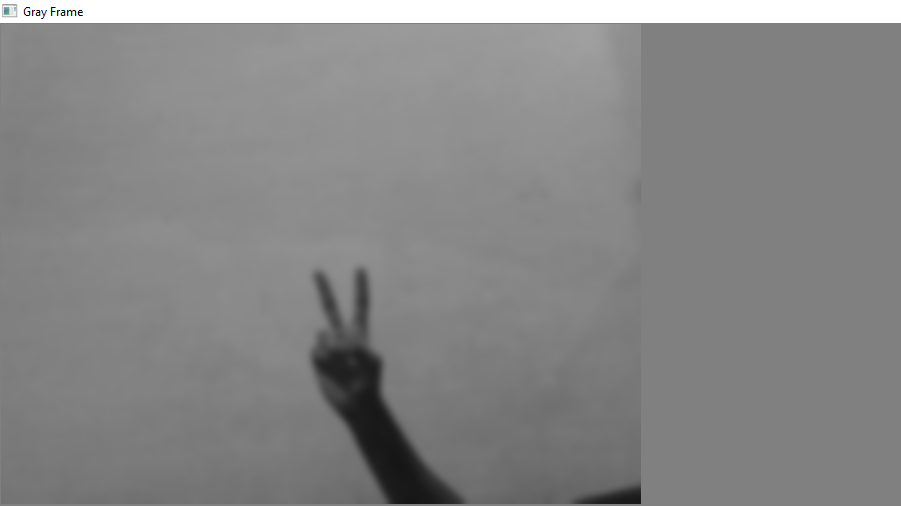2. Difference Frame : Difference frame shows the difference of intensities of first frame to the current frame.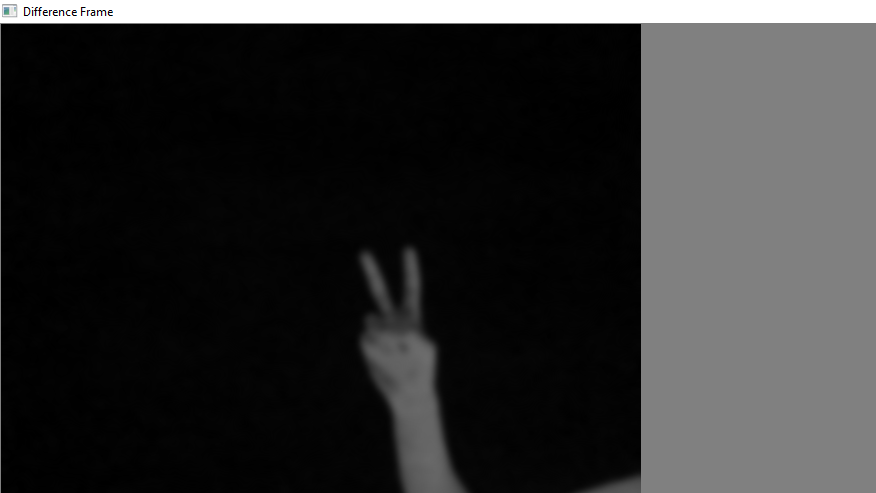3. Threshold Frame : If the intensity difference for a particular pixel is more than 30(in my case) then that pixel will be white and if the difference is less than 30 that pixel will be black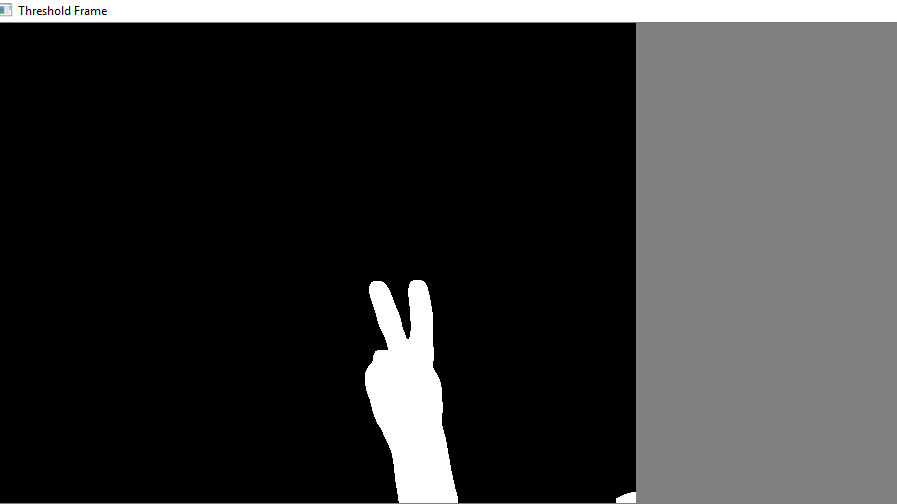4. Color Frame : In this frame you can see the color images in color frame along with green contour around the moving objects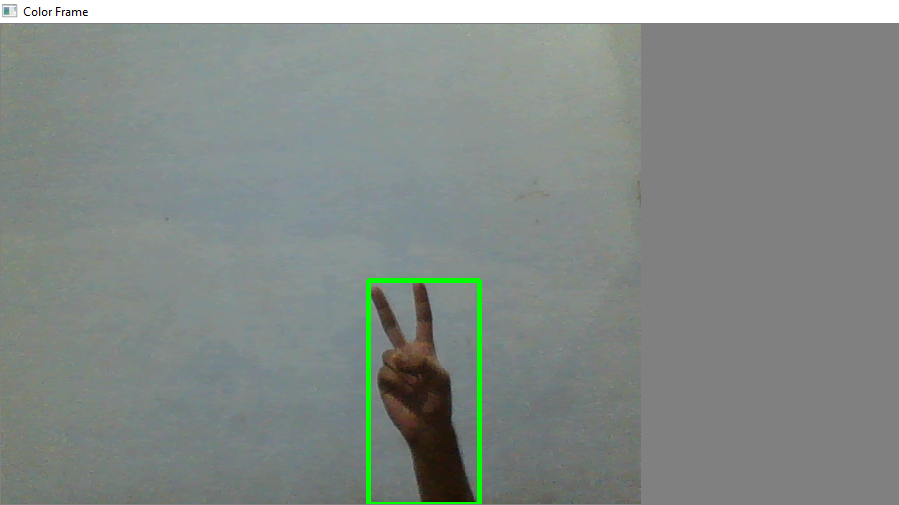Time Record of movements

The Time_of_movements file will be stored in the folder where your code file is stored. This file will be in csv extension. In this file the start time of motion and the end time of motion will be recorded. As you can see in picture: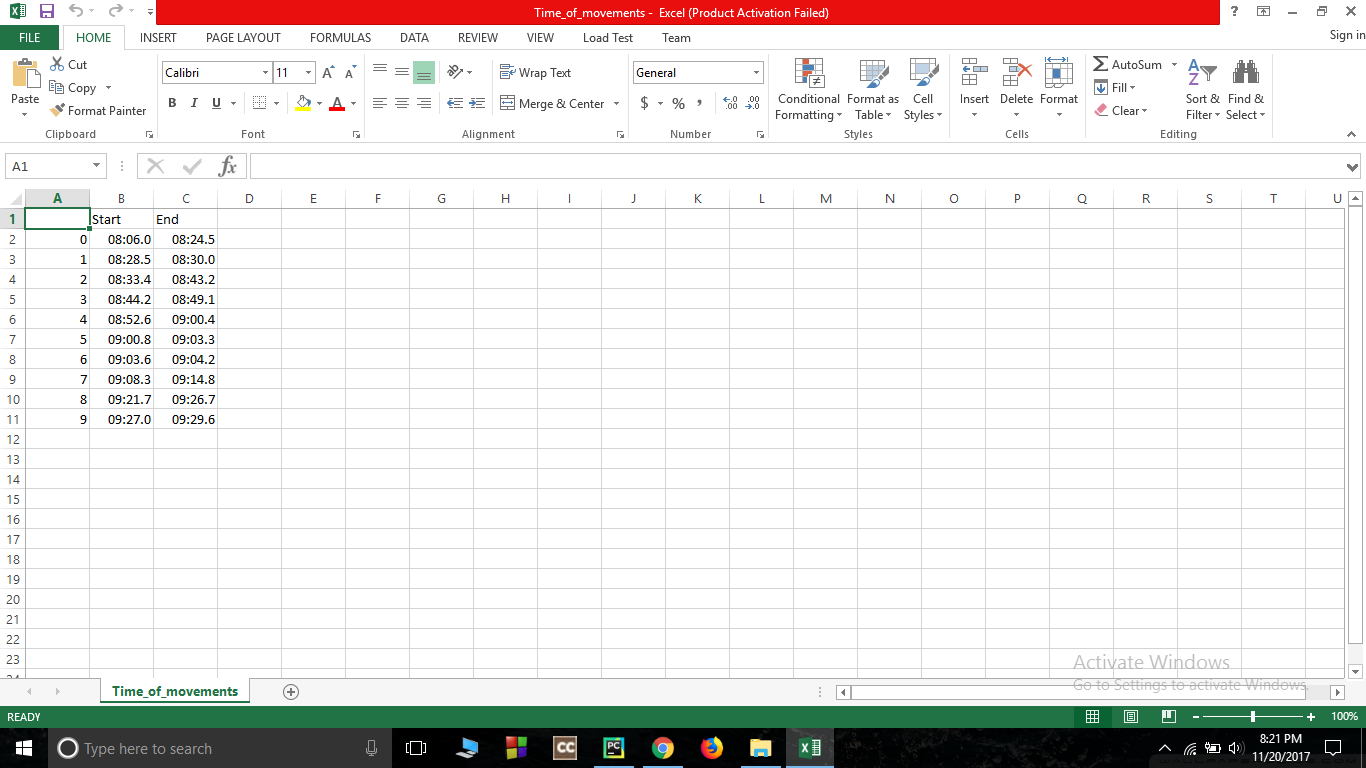Video Demonstration

My Personal Notes arrow_drop_upCheck out this Author's contributed articles.

If you like GeeksforGeeks and would like to contribute, you can also write an article using contribute.geeksforgeeks.org or mail your article to contribute@geeksforgeeks.org. See your article appearing on the GeeksforGeeks main page and help other Geeks.

Please Improve this article if you find anything incorrect by clicking on the "Improve Article" button below.

Improved By : nidhi_biet